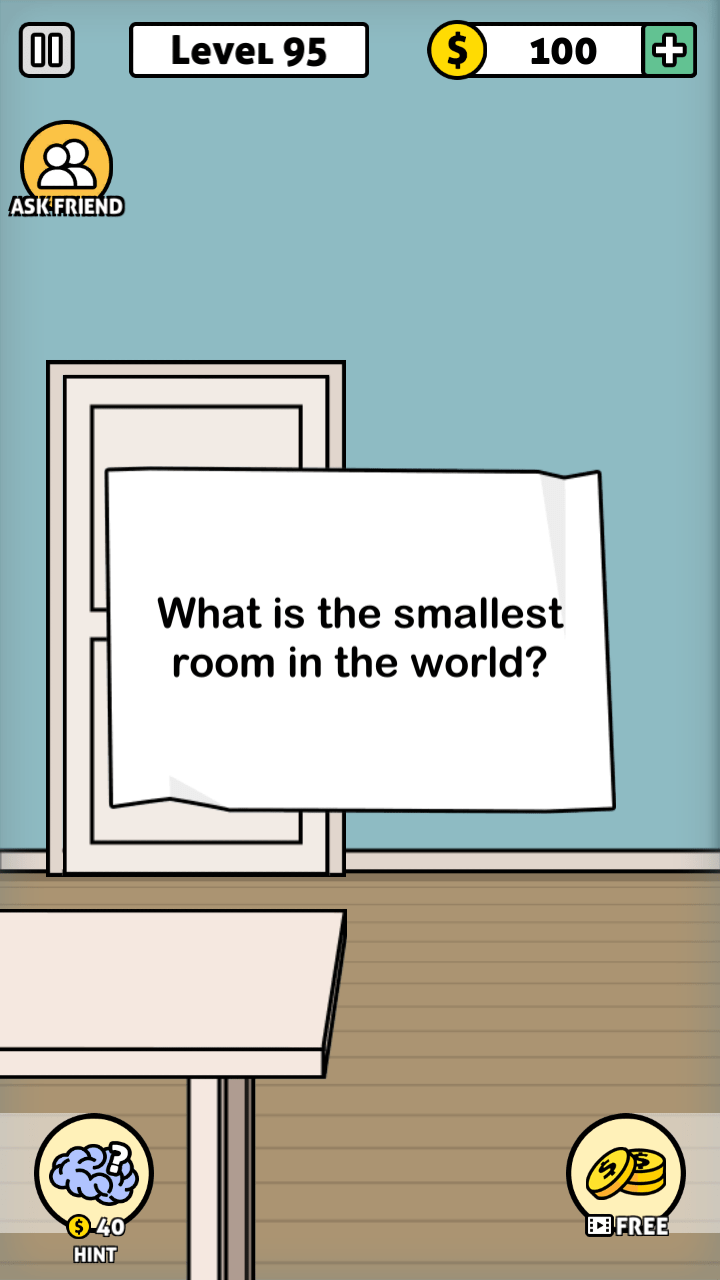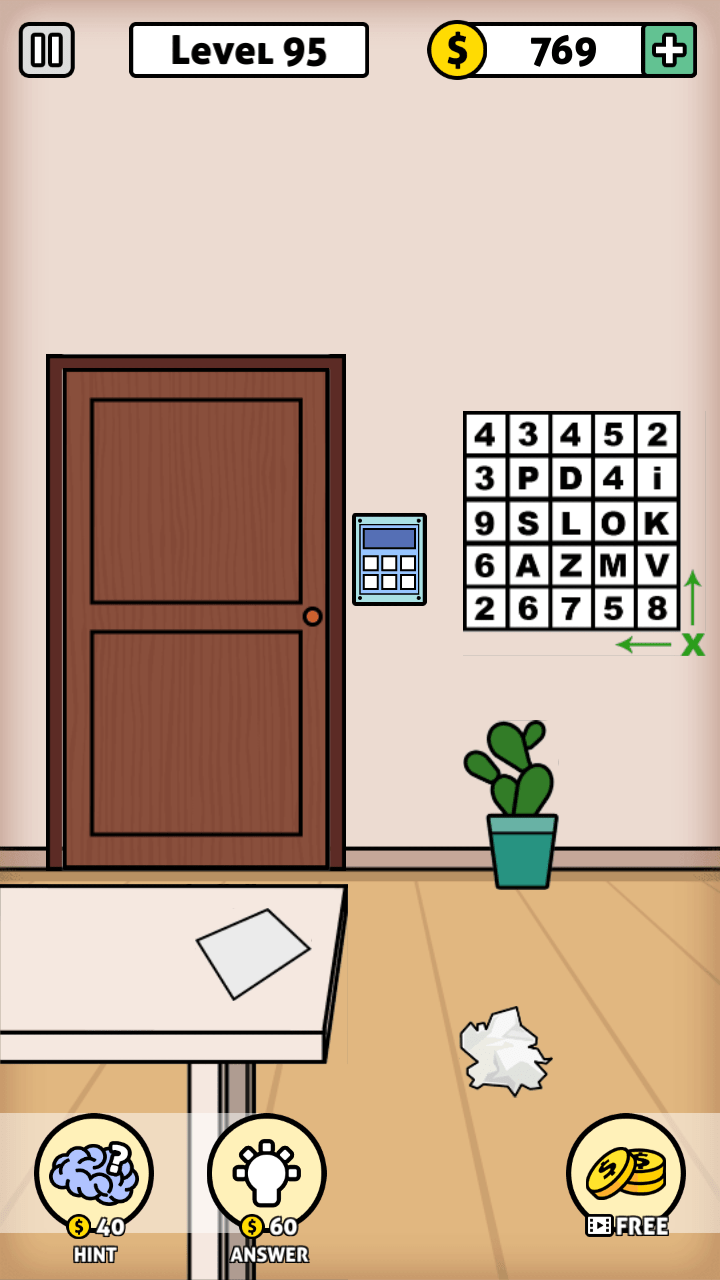Escape Room: Mystery Word Level 95 Answers

After every update we share here answer and photo of Level 95 of Escape Room: Mystery Word Game. Enjoy!If your picture and answer are different at our main page we collected and shared all versions answers for Game. Please check all answers for Game here Escape Room: Mystery Word Answers and Cheats

1. Hi regarding escape room level 95 I got the answer is sixty, but why??
The coloured letters are ali, don’t understand the grid

• The only way I could get to this answer using the table was to take the numbers at the top of the column and left of the row for each letter, multiply those together to get the value of each letter, then add the 3 results together. So here you go…

chart:
4 3 4 5 2
3 P D 4 i
9 S L O K
6 A Z M V
2 6 7 5 8

A = 6 * 3 = 18
L = 9 * 4 = 36
i = 8 * 3 = 6
A + L + i = 60

2. EscapeRoom Mystery Word Level 95
I don’t get your interpretation of the grid
Can you explain how you got the L=9: I=8
And where you got the numbers you multiplied with

3. I didnot understand how you got the numbers

4. The X with arrows pointing left and up indicates the numbers to multiply are to the left and the above of the letter, in this case A, L and i.

Find A and go to the left, you find 6 on the leftmost row. Now go up from A, you find 3 on the top row.
Find L and go to the left, you find 9 on the leftmost row. Back to L and go up, you find 4 on the top row.
Find i and go to the left, you find 3 on the leftmost row. Back to i and go up, you find 2 on the top row.

Peter’s explanation is correct, but has one typo. i = 8 * 3 = 6 should be i = 3 * 2 = 6.

5. The four in the second row should be a letter. This confused me as I tried to mutiply it in the answer. Also the bottom row of digits should be removed as they cause confusion, as I suspect a number of people would agree! !